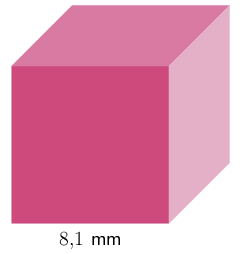Home Practice
For learners and parents For teachers and schools
Textbooks
Full catalogue
Pricing SupportLog in

We think you are located in United States. Is this correct?

# Solving problems with exponents and roots

## 3.5 Solving problems with exponents and roots

We work with the power of $$3$$ when we find the volume of a cube. To find the side of a cube if the volume is given, we need to take the cube root of the volume.

The volume of a cube is given by the formula

$V = s^{3}$

where $$s$$ is the side length of the cube.

Therefore, $$s = \sqrt{V}$$.

## Worked example 3.9: Find the volume of a cube

Calculate the volume of the following cube. Round your answer to two decimal places.### Use the formula for the volume of a cube.

$$V = s^{3}$$

### Substitute the given side value, $$\mathbf {s = \text{8,1}}$$.

$$V = (\text{8,1})^{3} = \text{531,441} \text{ mm}^{3}$$

### Now, round the answer to two decimal places.

$$\text{531,44} \text{ mm}^{3}$$

## Worked example 3.10: Find the volume of a cube

Given: the volume of a cube, $$V = 27 \text{ cm}^{3}$$. Find the side of this cube, in centimetres.

To find the side of a cube with the given volume, we take the cube root of the volume:

$s = \sqrt{V}$

So, $$s = \sqrt{27} = 3\text{ cm}$$.

temp text